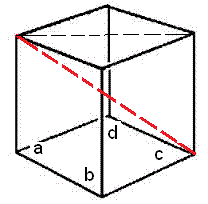Cube Calculator

Calculate the volume of cube, surface area of cube and diagonal of cube for given values:

Volume of Cube: [ ]

 Enter the Length : Volume of Cube =

Surface Area of Cube: [ 6a² ]

 Enter the Length = Surface Area of Cube =

Diagonal of Cube: [ Sqrt(3)*a ]

 Enter the Length = Diagonal of Cube =

The Cube is regular hexahedron having six faces which are all squares, the each face has four equal sides and all four interior angles are right angles. The cube has 12 edges and 8 vertices.
On putting the value a for the length, width and height into the formulas for volume and surface area of a rectangular solid.
See Volume and Surface Area of a Cube, below:
Volume of cube : a x a x a = a3
Surface Area of Cube: [ 6a² ]
Diagonal of Cube: [ √3 a ]Diagonal of Cube ?

The diagonal of a cube is a line segment that joins two vertices of a cube. It is shown with red line in figure.

Properties of a Cube

A cube is a three-dimensional solid object having different diagonals of a cube as the face diagonals and the body diagonal. It has the equal length, width, and height and each of its faces is a square:

The cube has 12 edges, 6 faces, and 8 vertices.

All the faces of a cube are having square shaped, therefore the length, breadth and height are the same.

Each of the faces in a cube meets the other four faces.

The opposite faces or planes in a cube are parallel to each other.

The opposite edges in a cube are parallel to each other.

The angles between any two faces or surfaces are 90°.

Each of the vertices in a cube meets the three faces and three edges.